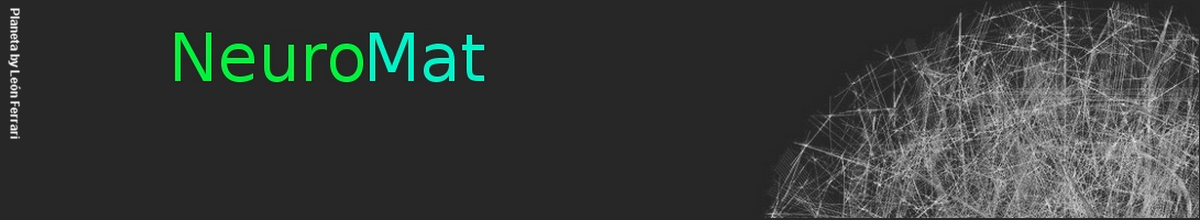### Independence tests for continuous random variables based on the longest increasing subsequence

by García, J. E. and González-López, V. A.

We propose a new class of nonparametric tests for the supposition of independence between two continuous random variables X and Y. Given a size n sample, let π be the permutation which maps the ranks of the X observations on the rank of the Y observations. We identify the independence assumption of the null hypothesis with the uniform distribution on the permutation space. A test based on the size of the longest increasing subsequence of π (Ln) is defined. The exact distribution of Ln is computed from Schensted’s theorem [C. Schensted. Longest increasing and decreasing sub-sequeces. Canad. J. Math. 13 (1961) 179–191]. The asymptotic distribution of Ln was obtained by Baik et al. [J. Baik, P. Deift, K.Johansson. On The Distribution of the Length of the Longest Increasing Subsequence of Random Permutations. J. Amer. Math. Soc. 12 (1999) 1119–1178]. As the statistic Ln is discrete, there is a small set of possible significance levels. To solve this problem we define the JLn statistic which is a jackknife version of Ln, as well as the corresponding hypothesis test. A third test is defined based on the JLMn statistic which is a jackknife version of the longest monotonic subsequence of π. On a simulation study we apply our tests to diverse dependence situations with null or very small correlations where the independence hypothesis is difficult to reject. We show that Ln, JLn and JLMn tests have very good performance on that kind of situations. We illustrate the use of those tests on two real data examples with small sample size.

The whole paper is available here: www.sciencedirect.com/science/article/pii/S0047259X14000335

NeuroCineMat
Featuring this week: